# Class 1: Basics, variables, operators

## Basics

MEL characteristics:

• free-form
• whitespace - spaces, tabs, blank lines
• scripts are made up of 'procedures' which are made up of 'statements'
• semi-colons terminate statements
• comments are in two flavors: // and /* .. */
```
/* this is a comment
that spans multiple lines */

// this is a one liner

```

Throughout these pages of notes, what's shown against a light background is MEL code which you can copy and paste verbatim into your script ed. window, eg:

```
sphere; // create a NURBS sphere..

```

## Variables

Variables form the currency of modern programming:

• placeholders for data [memory locations]
• just two operations - get, "put"
• name, type and a value
• \$..
• proper naming is essential to maintainability

Exactly five built-in variable types in MEL:

• int
• float
• string
• vector
• matrix

Of the above five types, only int, float, string and vector types can be arrays (see below). So MEL has a total of 9 built-in variable types: 5 'primitive' ones, 4 array ones. NO MORE! In other words, new var. types CANNOT be added (no 'structs' or 'classes').

## integer variables

```
int \$i; int \$j=5;
int \$k, \$l;
int \$i = 4.765; // will be truncated (NOT rounded) to 4

```

## float variables

```
float \$f1;
float \$f1a, \$f1b;
float \$f2 = 6.65;
float \$f3 = 1/3; // \$f3 will be 0, NOT 0.3333333
float \$f4 = 1.0/3; // will be 0.333333333 as expected

```

## string variables

```
string \$a;
string \$fnm = "Los ";
string \$lnm ="Angeles";
// concatenation; also note the use of a space character
string \$fullName = \$fnm + " " + \$lnm;

```

## vector variables

```
vector \$v1 = <<1.0,0.56,-0.96>>;
print(\$v1.x); // NEED the ()
\$v1 = <<\$v1.x,\$v1.y,10.0>>; // NEED to reset all values, can't just set \$v1.z [see below]
\$v1.z = 10.0; // NOT allowed, syntax error

```

## matrix variables

```
matrix \$n;
matrix \$i2 = <<1,2,3;-6,.78,.45>>; // 2 rows,3 columns. Note the use of ';' to separate rows
\$j2 = \$i2; // \$j2 is another matrix created with same contents as \$i2 [copied over]
\$k2 = -\$i2; // \$k2 is a new matrix with values from \$i2 NEGATED and copied over

```

• can +,-,*,/and % a matrix by a scalar [float or int]
• rows are separated by semicolons, columns by commas
• multiplication of matrices yields a new result matrix
• can negate a matrix
• indices start at 0, not 1
• to access an individual element, say '\$f = \$m`,etc.
• are not at all widely used :)

Deformations such as twist, taper and bend can be expressed in the form of matrices. Every vertex/CV of a shape can then be multiplied with such matrices to yield deformed shapes.

## arrays

• ordered collections of primitive types [except matrices]
• used for lists, grouping data..
• can grow as needed [dynamic memory allocation]
• size(\$arrayname)
• clear() to delete
• access: [] to specify an index, eg. '\$nextNum = \$hugeArray;'
• indices start at 0, not 1
```
int \$a2[];
int \$b2;
// here's how to initialize an array [specify all values while creating the variable]
float \$flArr= {0.8,-.6,1.,14.,12.3,-7.6};

clear \$b2; // empty out contents, size goes to 0
\$b2 = 1969; // this assignment will grow the size to 10000

```

## Operators

• symbols ["punctuation", informally speaking] that work with keywords and variables to form expressions
• for example, the assignment operator is '='

## arithmetic operators

```
+
-
*
/
%

+=
-=
*=
/=
++ [equivalent to +=1]
-- [equivalent to -=1]
Note: there's no [need for a] '**' or '//' operator!

```

## relational [comparison] operators

These express relationships between two variables' contents (ie. values they contain).

```
==
!=
>
<
>=
<=

```
```
// The result of a comparison is captured in an int variable. Eg.
int \$areTheyIdentical = (\$a==\$b); // are 'a' and 'b' identical?
```

## logical operators

The three operators AND (&&), OR (||) and NOT (!) implement "Boolean" logic. These are HEAVILY used in loops and branches to express program logic, so you do need to understand these very, very well! Without logical operators, modern computer programming simply cannot exist.

In the following "truth tables", A and B are 'tests' (questions) where their answer is always a yes (1) or no (0).

```
&& (AND, or 'intersection')
------------------
A B    A_AND_B
------------------
0 0    0
1 0    0
0 1    0
1 1    1
"A AND B" is true *ONLY IF* A is true and also B is true.

|| (OR, or 'union')
-----------------
A B    A_OR_B
-----------------
0 0    0
1 0    1
0 1    1
1 1    1
"A OR B" is true *IF EITHER* A is true or B is true (or both are true).

! (NOT, or 'inversion')
-------------
A    NOT_A
-------------
0    1
1    0
'NOT A' is the opposite of A (duh!!).
```

Interestingly, as per "de Morgan's laws", we can use the pair (AND, NOT) to replace OR [likewise, the pair (OR, NOT) to replace AND]:

```
'A OR B' identity (OR is expressed purely in terms of AND, NOT):
NOT(NOT(A) AND NOT(B)) = NOT(NOT(A)) OR NOT(NOT(B)) = A OR B

'A AND B' identity (AND is expressed purely in terms of OR, NOT):
NOT(NOT(A) OR NOT(B)) = NOT(NOT(A)) AND NOT(NOT(B)) = A AND B

```

In the above, A and B are tests, eg. A can be (\$a==\$c) and B can be (\$a>\$d). Using these as examples, the 'A AND B' identity above means this:

```
int \$b;
\$b = (\$a==\$c) && (\$a>\$d); // A AND B
\$b = !( !(\$a==\$c) || !(\$a>\$d) ); // NOT( NOT(A) || NOT(B) )

```

If you create variables \$a, \$b, \$c, \$d, provide values for \$a, \$c and \$d, then run the above two MEL expressions, you'll find that \$b comes out to be the same in both cases (both will come out 1 or come out 0). Doing verifies the 'A AND B' identity for us.

So if the && operator is banished from MEL (or from all programming languages in the world!) we can make do with ||. Likewise, if || goes away, we can use && instead. But ! CANNOT go away, it is ESSENTIAL. In other words "you cannot not use NOT!".

Exercise 1: use truth tables to prove the above two identities.

Exercise 2: verify the 'A OR B' identity above, using MEL, just like we did for 'A AND B'.

The &&, II and ! operators are related to logical circuits (the fundamental building blocks of computation!) and to set theory.

Here are the three logic "gates" [regular and IEC (International Electrotechnical Commission) symbols, truth table]: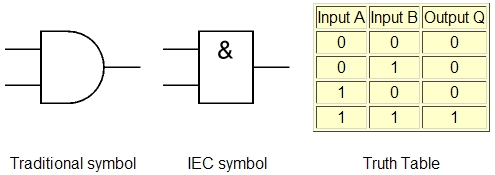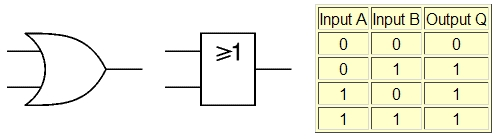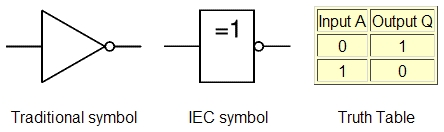Likewise, these are the set operations: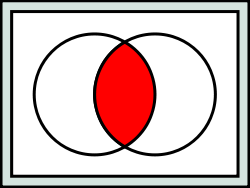For sets A and B (circles), their 'intersection' (shown in red) is equivalent to && - elements in the red area are in A *and* in B.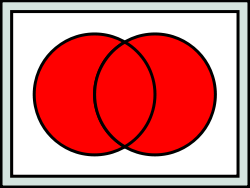The || operator is equivalent to set 'union' - an element can be in A *or* in B to be in the union set.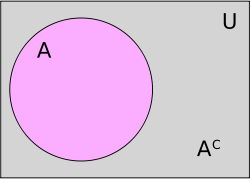The ! operator is like a set's complement (inversion) - it denotes elements (in gray) *not* in the set.